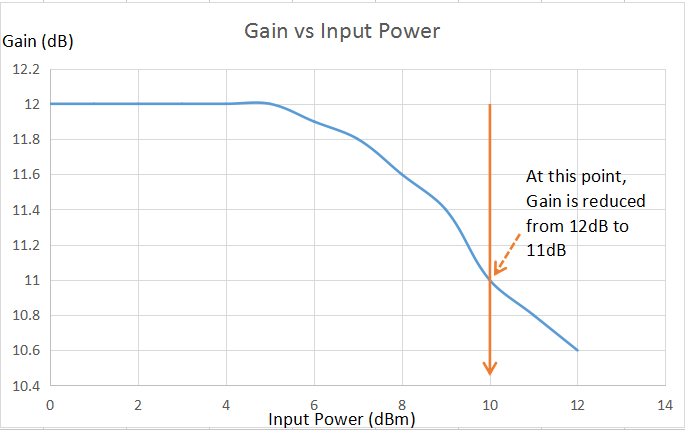# 1dB Compression Point (P1dB Point)

When operating in the linear region of a device (such as power amplifier, mixer etc.), gain through that device is constant. As the input power is increased, a point is reached when the output power of the device is not increased by the same amount as of input signal power, means there starts the compression of power at the output. A point where 1dB power is compressed at the output is known as 1dB compression point. In simple terms, if we plot the gain as a function of input power, we identify the point where the gain has dropped by 1dB. This is the 1dB compression point.

Gain compression occurs because eventually the output signal (voltage, current, power) limits, due to the supply voltage or bias current.

One general point about P1dB measurements that you should know: if you are working on a transmitter chain (or power amplifier), it is important to specify the P1dB point at the output (abbreviated P1dBout).As seen from the above graph, at input power level of 10dBm, one dB compression point occurs, so

Input P1dB point  = 10dBm.

Output P1dB point = Input P1dB point + Gain

Output P1dB point = 10 (input power) + 11 (gain at compression point)

= 21dBm

Quite often, microwave engineers refer to the 1-dB compression point, but 2 or 3 dB compression points are also important in power amplifier chains.

Tagged